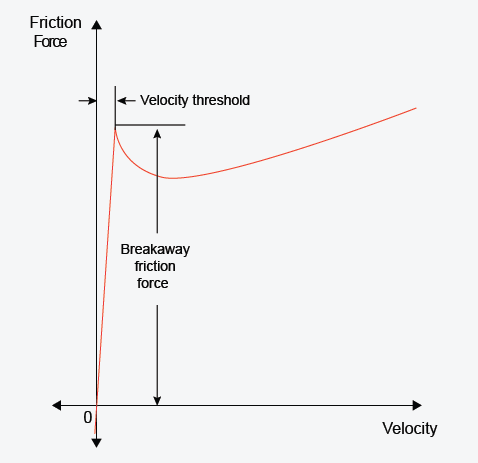# Translational Friction

Friction in contact between moving bodies

• Library:
• Simscape / Foundation Library / Mechanical / Translational Elements

•## Description

The Translational Friction block represents friction in contact between moving bodies. The friction force is simulated as a function of relative velocity and is assumed to be the sum of Stribeck, Coulomb, and viscous components, as shown in the following figure.The Stribeck friction, FS, is the negatively sloped characteristics taking place at low velocities . The Coulomb friction, FC, results in a constant force at any velocity. The viscous friction, FV, opposes motion with the force directly proportional to the relative velocity. The sum of the Coulomb and Stribeck frictions at the vicinity of zero velocity is often referred to as the breakaway friction, Fbrk. The friction is approximated with the following equations:

`$F=\sqrt{2e}\left({F}_{brk}-{F}_{C}\right)\cdot \mathrm{exp}\left(-{\left(\frac{v}{{v}_{St}}\right)}^{2}\right)\cdot \frac{v}{{v}_{St}}+{F}_{C}\cdot \mathrm{tanh}\left(\frac{v}{{v}_{Coul}}\right)+fv$`
`${v}_{St}={v}_{brk}\sqrt{2}$`
`${v}_{Coul}={v}_{brk}/10$`
`$v={v}_{R}-{v}_{C}$`

where

• F is friction force.

• FC is Coulomb friction.

• Fbrk is breakaway friction.

• vbrk is breakaway friction velocity.

• vSt is Stribeck velocity threshold.

• vCoul is Coulomb velocity threshold.

• vR and vC are absolute velocities of ports R and C, respectively.

• v is relative velocity.

• f is viscous friction coefficient.

The exponential function used in the Stribeck portion of the force equation is continuous and decays at velocity magnitudes greater than the breakaway friction velocity.

The hyperbolic tangent function used in the Coulomb portion of the force equation ensures that the equation is smooth and continuous through v = 0, but quickly reaches its full value at nonzero velocities.

The block positive direction is from port R to port C. This means that if the port R velocity is greater than that of port C, the block transmits force from R to C.

### Variables

To set the priority and initial target values for the block variables prior to simulation, use the Initial Targets section in the block dialog box or Property Inspector. For more information, see Set Priority and Initial Target for Block Variables.

Nominal values provide a way to specify the expected magnitude of a variable in a model. Using system scaling based on nominal values increases the simulation robustness. Nominal values can come from different sources, one of which is the Nominal Values section in the block dialog box or Property Inspector. For more information, see Modify Nominal Values for a Block Variable.

## Ports

### Conserving

expand all

Mechanical translational conserving port associated with the rod, that is, the moving body.

Mechanical translational conserving port associated with the case, that is, the stationary body.

## Parameters

expand all

The breakaway friction force, which is the sum of the Coulomb and the static frictions. It must be greater than or equal to the Coulomb friction force value.

The velocity at which the Stribeck friction is at its peak. At this point, the sum of the Stribeck and Coulomb friction is the Breakaway friction force. This parameter specifies the velocity threshold, which affects the tradeoff between the simulation accuracy and speed.The Coulomb friction force, which is the friction that opposes motion with a constant force at any velocity.

Proportionality coefficient between the friction force and the relative velocity. The parameter value must be greater than or equal to zero.

 Armstrong, B. and C.C. de Wit, Friction Modeling and Compensation, The Control Handbook, CRC Press, 1995.